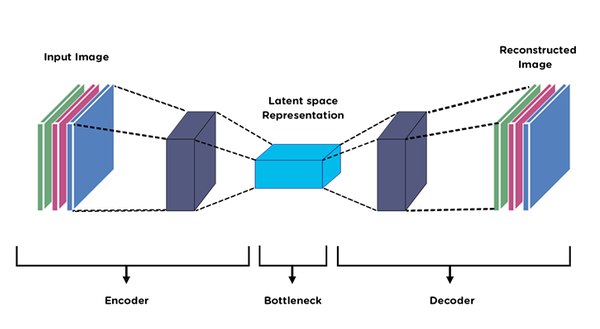# Autoencoders

Contents

A blog post explaining the machine learning-premise of my summer research at Oak Ridge National Lab.

Definition: An autoencoder is a neural network that is trained to copy its input to its output.

An autoencoder consists of two parts:

1. An encoder that produces a latent space representation from input data.
2. A decoder that recreates the input data from the latent space representation.

The latent space representation is a new representation of the underlying input data, typically with desirable properties such as having a lower dimension. This allows it to learn useful features about the data. Typically, autoencoders are trained only to approximately copy data and not to perfectly copy data.

## 1 Undercomplete Autoencoders

The most typical way to create an autoencoder is to have the encoder dimension have a smaller dimension than the data, creating a bottleneck.

An autoencoder whose code dimension is less than the input dimension is called undercomplete. Learning an undercomplete representation forces the autoencoder to capture the most salient features of the training data.A visual representation of the structure of an autoencoder. The goal is typically not to exactly reconstruct the original image, but rather to learn useful features and represent them in the latent space representation.

The learning process consists of optimizing a loss function that represents how similar the reconstructed output is to the input.

$$L(x, g(f(x))) \ \text{Example: } L(x, g(f(x)) = ||\vec{x} - g(f(\vec{x}))||$$

One can show that using a mean-squared error loss function results in a powerful nonlinear generalization of PCA (provided that $f$ and $g$ are nonlinear).

## 2 Regularized Autoencoders

Instead of reducing the dimension (or perhaps alongside reducing the dimension) of the code dimension, one can introduce a regularization term that “punishes” the model for copying input.

### Sparse Autoencoders

A sparse autoencoder is an autoencoder that encourages the model to create a sparse code representation of the input; i.e. use as few variables as possible to represent the underlying input data.

• This corresponds to a lower code dimension.

This involves modifying the loss function to include a sparse penalty on the code layer $h$:

$$L(x, g(f(x))) + \Omega(h)$$

These models are often used in conjuction to other models. A sparse autoencoder performs dimensionality reduction on data, which can then be fed into another model (e.g. classifier).

## 3 Denoising Autoencoders

Instead of providing an original image or data $x$ as input to a decoder, we can instead add noise to the image or data and feed $\tilde{x}$ into the autoencoder. The decoder tries to reconstruct the original image from the corrupted image.

The autoencoder now tries to optimize the loss function

$$L(x, g(f(\tilde{x})))$$

## 4 Applications

The most common application of autoencoders is to perform dimensionality reduction. Higher dimensional datasets often suffer from the curse of dimensionality, where the feature space is too large to have enough data points to fully learn.

By using an autoencoder, the code serves as a lower dimensional representation of the same data, which can then be easily learned.

Sparse autoencoders in particular lend themselves to highly interpretable models, where each element in the code representation tends to control one easily observable feature.

### My Research

My research at Oak Ridge National Lab consists of performing this autoencoding step. My goal is to find an efficient representation of neutron scattering spectra using an autoencoder. Then, I would feed this representation into a pre-existing model to predict 2- and 3-dimensional spectra from crystal structure.

Currently, a model to predict a 1-dimensional spectrum from crystal structure exists and is highly effective, but no such model exists for higher dimensions. Furthermore, ab initio calculations of the neutron scattering spectra (such as via DFT) are extremely slow and require incredibly large computational capabilities. This data is extremely useful to researchers who work with neutron scattering spectra, which can be used to determine properties (e.g. heat capacity, vibrational modes) of crystals and other materials.